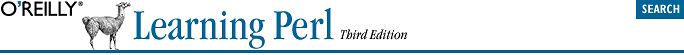home | O'Reilly's CD bookshelfs | FreeBSD | Linux | Cisco | Cisco Exam## 10.7. The for Control Structure

```for (initialization; test; increment) {
body;
body;
}```
```initialization;
while (test) {
body;
body;
increment;
}```

The most common use of the for loop, by far, is for making computed iterations:

```for (\$i = 1; \$i <= 10; \$i++) {  # count from 1 to 10
print "I can count to \$i!\n";
}```

When you've seen these before, you'll know what the first line is saying even before you read the comment. Before the loop starts, the control variable, \$i, is set to 1. Then, the loop is really a while loop in disguise, looping while \$i is less than or equal to 10. Between each iteration and the next is the increment, which here is a literal increment, adding one to the control variable, which is \$i.

So, the first time through this loop, \$i is 1. Since that's less than or equal to 10, we see the message. Although the increment is written at the top of the loop, it logically happens at the bottom of the loop, after printing the message. So, \$i becomes 2, which is less than or equal to 10, so we print the message again, and \$i is incremented to 3, which is less than or equal to 10, and so on.

Eventually, we print the message that our program can count to 9. Then \$i is incremented to 10, which is less than or equal to 10, so we run the loop one last time and print that our program can count to 10. Finally, \$i is incremented for the last time, to 11, which is not less than or equal to 10. So control drops out of the loop, and we're on to the rest of the program.

All three parts are together at the top of the loop so that it's easy for an experienced programmer to read that first line and say, "Ah, it's a loop that counts \$i from one to ten."

Obligatory This is Spinal Tap outdated pop-culture reference.

Of course, it never gets to 1000 exactly. The last iteration uses 999, since each value of \$i is a multiple of three.

```for (\$i = -150; \$i <= 1000; \$i += 3) {
print "\$i\n";
}```

In fact, you could make any of the three control parts (initialization, test, or increment) empty, if you wish, but you still need the two semicolons. In this (quite unusual) example, the test is a substitution, and the increment is empty:

```for (\$_ = "bedrock"; s/(.)//; ) {  # loops while the s/// is successful
print "One character is: \$1\n";
}```

The test expression (in the implied while loop) is the substitution, which will return a true value if it succeeded. In this case, the first time through the loop, the substitution will remove the b from bedrock. Each iteration will remove another letter. When the string is empty, the substitution will fail, and the loop is done.

If the test expression (the one between the two semicolons) is empty, it's automatically true, making an infinite loop. But don't make an infinite loop like this until you see how to break out of such a loop, which we'll discuss later in this chapter:

```for (;;) {
print "It's an infinite loop!\n";
}```

If you somehow made an infinite loop that's gotten away from you, see whether Control-C will halt it. It's possible that you'll get a lot of output even after typing Control-C, depending upon your system's I/O and other factors. Hey, we warned you.

```while (1) {
print "It's another infinite loop!\n";
}```